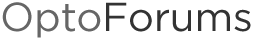# Round Command Upgrade Request

Can we upgrade the round command to function more like VB and Excel? I think it is useful to be able to specify significant digits.

PAC Control Round:

VB Round:

Excel Round:

Yes, it would be nice if it was built in. You could put the following in a subroutine. If you only want to round after the decimal point, then it can be reduced to a couple lines.

``````//Value is the float that you want to round
//DecimalPlace is an integer of the decimal place you want to round to, negative numbers work too
//Decimal place must be in the range -7..7 due to floating point precision

if(DecimalPlace == 0) then
Value = Round(Value);
else
if(DecimalPlace < 0) then  //Opto Power function only works with positive exponents!!!
fMultiplier = 1 / Power(10, AbsoluteValue(DecimalPlace));
else
fMultiplier = Power(10, DecimalPlace);
endif

Value = Round(Value * fMultiplier) / fMultiplier;
endif``````

Hi philip,
Thanks for the nice sample code.
I was surprised to see your comment on “positive exponents.” I see that in the doc, but I tried it with -1 and it worked fine, so perhaps that doc note is old, I’ll get it updated.
Thanks again,
Mary

Hi Mary,

I wrote the code in 9.3, and it would not work with negative exponents as the documentation states. There must of been an update since then.

Philip

Okay, I must of read the docs before testing it - I may have been ill.The negative exponents do work in 9.3, so the code can be simplified to the following:

``````fMultiplier = Power(10, DecimalPlace);
Value = Round(Value * fMultiplier) / fMultiplier;``````
1 Like